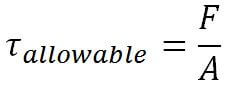# How to Determine the Shear Strength of a Fillet Weld

In our previous post we explained how to determine the strength of a transverse fillet weld.  A transverse fillet weld is one that is perpendicular to an applied load.  We will now look at how to determine the strength of a fillet weld under shear stress.

If the load applied is not perfectly perpendicular to the fillet weld, the weld is in shear and its load carrying capacity is greatly reduced.   Because of this reason, when designing welds we always assume that the weld will be loaded in shear as seen below.

In this case the applied load is parallel to the welds.  The forces are pulling the members being joined in opposite directions, which places the welds under shear.  When a weld is in shear we cannot longer use the tensile strength of the filler metal to determine the strength of the weld.  Instead, the tensile strength is reduced by a factor in order to assure safety. Clause 2 of AWS D1.1 requires that the minimum tensile strength of the filler metal be multiplied by 0.30 to obtain the allowable shear stress on the weld.

The calculation is the exact same as when calculating the strength of a transverse fillet weld, except for the safety factor.  The notation now shows the maximum shear stress (τ) allowed rather than the maximum tensile stress (σ).Where,

is the maximum allowed shear stress on the weld

F is the force the weld can handle, in other words, the strength of the weld in lbf

A is the effective area of the weld

Notice that instead of  (tensile stress) we now have  (allowable shear stress).

For our example we will use the same numbers we used on our previous post for determining the strength of a transverse fillet weld.   We are using an ER70S-6 filler metal with minimum tensile strength of 70,000 psi.  We are placing two (2) 20-inch long lap welds on both sides of the joint.The allowable shear stress for the welds would be 70,000 psi x 0.30 = 21,000 psi.   A reduction of 70% compared to the case where the fillet weld was in pure tension.

If our two welds are ¼-inch fillets then the shear strength (load carrying capacity) of the welds is calculated as follows.

First, we rearrange the formula provided above to solve for F.We already know that the allowable shear stress is 70,000 x 0.30 = 21,000 psi.  To get A (effective area of the weld) we need to multiply the theoretical throat size (1/4 x 0.707 = 0.177 inches) times the length (20 inches) times 2 welds.

The effective area equals 20in x 0.177in x 2 = 7.08 sq-in.

We can now solve for F.

F = 21,000 x 7.08 = 148,680 lbf

Because the welds were placed parallel to the applied load, the allowable force is 70% less than if we had placed the welds perpendicular to the applied load (as in the example on the previous post).

Understanding how loads act upon weldments is not easy, even for accomplished welding engineers and design engineers.  But having this knowledge can improve structural integrity and reduce costs by reducing the amount of weld.

Please note: I reserve the right to delete comments that are offensive or off-topic.

## 5 thoughts on “How to Determine the Shear Strength of a Fillet Weld”

1.Sopian says:

Thank you, i need some knowledge on weld connections in structures.

•Welding Answers says:

What kind of information are you looking for? We get involved with welded connection design quite often. Anything specific you would like to know about?

2.dilanka says:

I want to know the factor of safety for fillet weld.

•Welding Answers says:

That would depend on the size of the fillet weld. You can design a weld to give you only the strength that is required to carry the load imposed on it. If the weld must be 3/16″ and you go with 1/4″ you will have a different safety factor than if you go with a 5/16″ fillet weld. The safety factor is determined by the design engineer that determines the weld sizes.